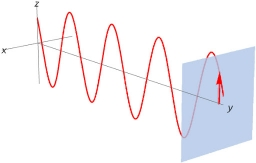# Oscillation 48961

Write the equation of harmonic oscillation if the amplitude of the oscillation is 5 cm and its period is 0.5 s.

Result

x = (Correct answer is: 5 * sin(ωt))### Step-by-step explanation:Did you find an error or inaccuracy? Feel free to write us. Thank you!

Tips for related online calculators
Most natural application of trigonometry and trigonometric functions is a calculation of the triangles. Common and less common calculations of different types of triangles offers our triangle calculator. Word trigonometry comes from Greek and literally means triangle calculation.